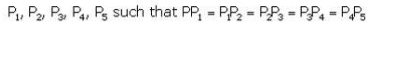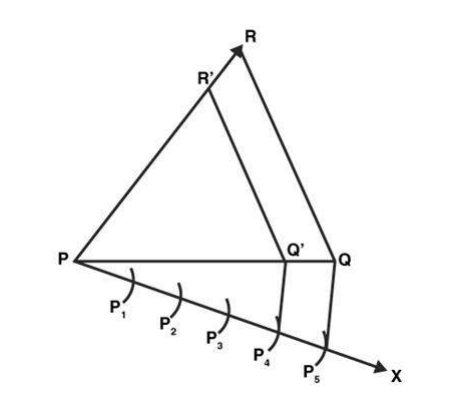# RS Aggarwal Solutions Class 10 Mathematics Solutions for Constructions Exercise 9A in Chapter 9 - Constructions

3: Construct a ∆PQR, in which PQ = 6 cm, QR = 7 cm and PR = 8 cm. Then, construct another a triangle whose sides are 4/5 times the corresponding sides of ∆PQR.

Steps of construction:

1. Draw a line segment PQ = 6 cm.
2. Draw an arc, using P as a center and radius = 8 cm
3. Draw another arc, using Q as a center and radius = 7 cm
4. Now, join PR and QR to get △PQR
5. Draw a ray PX by making an acute angle, angle QPX
6. Divide PX into 4 equal parts7.
7. Join P5Q
8. Draw a line P4Q' which is parallel to P5Q
9. Similar to step 8, draw a line Q’R’ which is parallel to QR10. Therefore, △PQ’R’ is the required triangle.
Related Questions

Lido

Courses

Teachers

Book a Demo with us

Syllabus

Maths
CBSE
Maths
ICSE
Science
CBSE

Science
ICSE
English
CBSE
English
ICSE
Coding

Terms & Policies

Selina Question Bank

Maths
Physics
Biology

Allied Question Bank

Chemistry
Connect with us on social media!